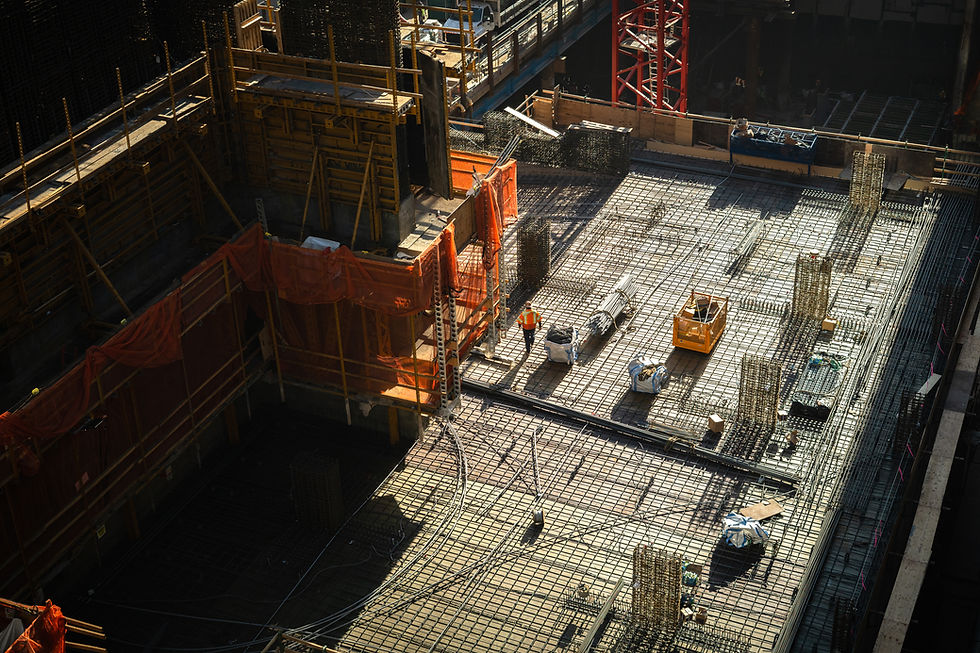top of page###### Simple Payback Period

Accounting (Year 12) - Capital Expenditure

Christian Bien

# What is the Payback Period and How is it Calculated?

The payback period is the time it takes to recover the cash outflows on an initial capital investment. For example, if you spent \$100 on a capital asset that generates \$10 each year, it will take 10 years to recover your money (breakeven).

Therefore, the Payback Period is 10 years. Therefore, for Capital Investments that generate a steady stream of cash per year, the Payback Period Formula is as follows: Payback Period = (Initial Cash Outflow) / (Annual Cash Inflows Per Year)

# Worked Example: Simple Payback Period

Below is an example of how to calculate the Payback Period for a capital investment that will generate the same amount of cash inflows each year. Chef Pty Ltd, requires an investment of a new kitchen which will cost \$100,000.

The Company estimates that the new kitchen will produce economic benefits of \$30,000 per year. Calculate the Payback Period for the Investment of the New Kitchen in Years and Months.

# Step 1: Calculate the Accumulated Cash Flows (Optional)

This step is for the purposes of explaining a concept - you can skip this step if required. We've extended the Payback Period table to include an additional column that calculates the accumulated cash flows - basically, the total cash flows added to date of that year.

E.g At Year 1 is \$30,000, at Year 2 is \$60,000 (\$30,000 Y1 + \$30,000 Y2). We can see there is a constant and same increase of \$30,000 in accumulative cash flow, therefore we can use the simple method of calculation of the Payback Period.

# Step 2: Apply the Payback Period Formula

As stated above, the formula for the Simple Calculation of the Payback Period is as follows: Payback Period = (Initial Cash Outflow) / (Annual Cash Inflows Per Year)

Therefore the Payback Period is: Payback Period = \$100,000 / \$30,000 = 3.33 years

However, we are asked to measure the payback period in months. We need to convert the decimals to months, to do this, get the decimal part (0.33 years) and multiply by 12.

0.33 years * 12 months = 3.96 months. Round Up to nearest whole number = 4 months

(Note: You ALWAYS round up, as if you round down, you technically haven't fully paid off the initial capital expenditure.)

Put the Answer Together You Get: = 3 Years + 4 Months. This makes sense as in the explanation image above, the 3.33 years sits between the low point at Year 3 at \$90,000, and the high point at \$120,000 at Year 4.

bottom of page